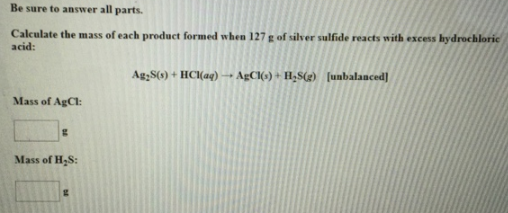# Problem: Be sure to answer all parts. Calculate the mass of each product formed when 127 g of silver sulfide reacts with excess hydrochloric acid: Ag2S (s) + HCl (aq) → AgCl (s) + H2S (s) [unbalanced] Mass of AgCl:Mass of H2S:

###### FREE Expert Solution
84% (377 ratings)###### Problem Details

Be sure to answer all parts.

Calculate the mass of each product formed when 127 g of silver sulfide reacts with excess hydrochloric acid:

Ag2S (s) + HCl (aq) → AgCl (s) + H2S (s) [unbalanced]

Mass of AgCl:

Mass of H2S: